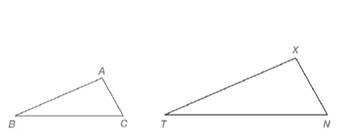Chapter 5.2, Problem 5E### Elementary Geometry for College St...

6th Edition
Daniel C. Alexander + 1 other
ISBN: 9781285195698

#### Solutions

Chapter
Section### Elementary Geometry for College St...

6th Edition
Daniel C. Alexander + 1 other
ISBN: 9781285195698
Textbook Problem
7 views

# In Exercises 5 and 6, refer to the drawing.a) Given that A ↔ X , B ↔ T , and C ↔ N , write a statement claiming that the triangles shown are similar.b) Given that A ↔ N , C ↔ X , and B ↔ T , write a statement claiming that the triangles shown are similar.Exercises 5, 6

To determine

a)

To write:

A statement claiming that the triangles shown are similar.

Explanation

Definition:

Similarity between Two polygons:

Two polygons are similar if and only if when all pairs of corresponding angles are congruent and all pairs of corresponding sides are proportional.

Given:

The triangles ABC and XTN are similar.

AX,BT,and CN.

Calculation:

According to the definition of similar polygons, the corresponding angles are congruent and corresponding sides are proportional

To determine

b)

To write:

A statement claiming that the triangles shown are similar.

### Still sussing out bartleby?

Check out a sample textbook solution.

See a sample solution

#### The Solution to Your Study Problems

Bartleby provides explanations to thousands of textbook problems written by our experts, many with advanced degrees!

Get Started

#### In Exercises 914, evaluate the expression. 11. (3 4)2

Applied Calculus for the Managerial, Life, and Social Sciences: A Brief Approach

#### In problems 9-18, simplify the expressions with all exponents positive. 18.

Mathematical Applications for the Management, Life, and Social Sciences

#### True or False: If F is conservative, then curl F = 0.

Study Guide for Stewart's Multivariable Calculus, 8th